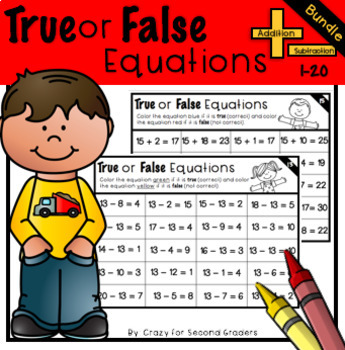# True or False Equations Addition and Subtraction Bundle1st - 3rd
Subjects
Standards
Resource Type
Formats Included
• Zip
Pages
86 pages
\$3.00
List Price:
\$4.00
You Save:
\$1.00
\$3.00
List Price:
\$4.00
You Save:
\$1.00

### Description

Save some money with this bundle!!!
Are you looking for a purposeful warm up or number sense activity?! LOOK NO FURTHER! This download is perfect for partner work, independent handouts, or centers. It's also a learning tool! Have students bring their page to a group or carpet time and have conversations about HOW they KNEW the answer was correct or incorrect. I love to hear how different all of their reasoning is!

Students are asked to look over the equations and determine if the answer is correct or incorrect. The download comes with a page for addition and subtraction 1-20 and the answers. 80 pages total.
Total Pages
86 pages
Included
Teaching Duration
N/A
Report this resource to TpT
Reported resources will be reviewed by our team. Report this resource to let us know if this resource violates TpT’s content guidelines.

### Standards

to see state-specific standards (only available in the US).
Add and subtract within 20, demonstrating fluency for addition and subtraction within 10. Use strategies such as counting on; making ten (e.g., 8 + 6 = 8 + 2 + 4 = 10 + 4 = 14); decomposing a number leading to a ten (e.g., 13 - 4 = 13 - 3 - 1 = 10 - 1 = 9); using the relationship between addition and subtraction (e.g., knowing that 8 + 4 = 12, one knows 12 - 8 = 4); and creating equivalent but easier or known sums (e.g., adding 6 + 7 by creating the known equivalent 6 + 6 + 1 = 12 + 1 = 13).
Understand the meaning of the equal sign, and determine if equations involving addition and subtraction are true or false. For example, which of the following equations are true and which are false? 6 = 6, 7 = 8 - 1, 5 + 2 = 2 + 5, 4 + 1 = 5 + 2.
Fluently add and subtract within 20 using mental strategies. By end of Grade 2, know from memory all sums of two one-digit numbers.

### Questions & Answers

Teachers Pay Teachers is an online marketplace where teachers buy and sell original educational materials.